Request a Tool

Cubic Meter Calculator

Cubic Meter Calculator is use to calculate cubic meters, centimeters, feets, inches, yards.

Cubic Meter
0

Formula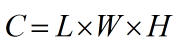• C = Cubic meter
• L = Value of length
• W = Value of width
• H = Value of height

Defination / Uses

The SI-derived unit of volume is the cubic metre or cubic metre. It is denoted by the symbol m3 in the International System of Units (SI). It's the volume of a one-metre-long cube.

Cubic Meter
0

Formula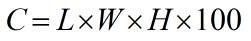• C = Cubic centimeter
• L = Value of length
• W = Value of width
• H = Value of height

Defination / Uses

A cubic centimetre is a volume measurement that equates to the volume of a cube of 1 cm x 1 cm x 1 cm. The volume of one millilitre is equal to one cubic centimetre. At 3.98 °C, the mass of one cubic centimetre of water is nearly equal to one gramme.

Cubic Meter
0

Formula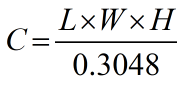• C = Cubic feet
• L = Value of length
• W = Value of width
• H = Value of height

Defination / Uses

In the United States and the United Kingdom, the cubic foot is an imperial and US customary unit of volume. It's defined as the volume of a cube with one-foot-long sides. It has a volume of 28.3168 litres. A cubic foot of water weighs 62.37 pounds at 60 degrees Fahrenheit.

Cubic Meter
0

Formula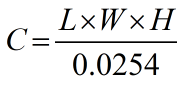• C = Cubic inch
• L = Value of length
• W = Value of width
• H = Value of height

Defination / Uses

The cubic inch is a volume unit used in both Imperial and US customary units systems. It is the volume of a cube whose three dimensions are each one inch long, equating to 1/231 of a US gallon.

Cubic Meter
0

Formula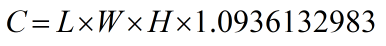• C = Cubic yard
• L = Value of length
• W = Value of width
• H = Value of height

Defination / Uses

In Canada and the United States, a cubic yard is an Imperial / US customary unit of volume. It's the volume of a cube with sides that are one yard long.

The SI derived unit of volume is the cubic metre (in Commonwealth English and international spelling as used by the International Bureau of Weights and Measures) or cubic metre (in American English). The SI symbol for it is m3. It's the volume of a cube with one-meter-long edges. The stère was an alternative term for dry measure that allowed for a different usage with metric prefixes. It is still occasionally used for dry measure (for instance, in reference to wood). The kilolitre was another possible moniker that is no longer regularly used. A cubic metre of pure water has a mass of 1000 kg, or one tonne, at maximum density (3.98 °C) and standard atmospheric pressure (101.325 kPa).